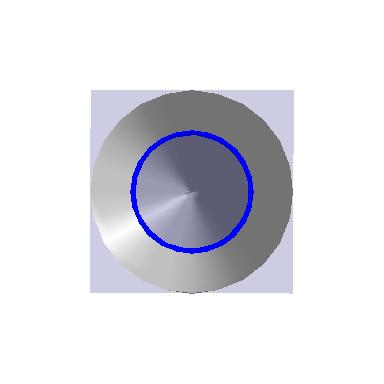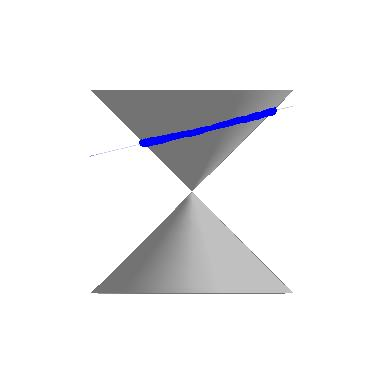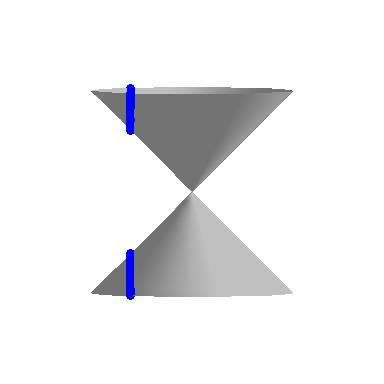$$\newcommand{\id}{\mathrm{id}}$$ $$\newcommand{\Span}{\mathrm{span}}$$ $$\newcommand{\kernel}{\mathrm{null}\,}$$ $$\newcommand{\range}{\mathrm{range}\,}$$ $$\newcommand{\RealPart}{\mathrm{Re}}$$ $$\newcommand{\ImaginaryPart}{\mathrm{Im}}$$ $$\newcommand{\Argument}{\mathrm{Arg}}$$ $$\newcommand{\norm}{\| #1 \|}$$ $$\newcommand{\inner}{\langle #1, #2 \rangle}$$ $$\newcommand{\Span}{\mathrm{span}}$$

# 7.1: Introduction to Conics

$$\newcommand{\vecs}{\overset { \rightharpoonup} {\mathbf{#1}} }$$

$$\newcommand{\vecd}{\overset{-\!-\!\rightharpoonup}{\vphantom{a}\smash {#1}}}$$

In this chapter, we study the Conic Sections - literally sections of a cone'. Imagine a double-napped cone as seen below being sliced' by a plane.If we slice the cone with a horizontal plane the resulting curve is a circle.Tilting the plane ever so slightly produces an ellipse.If the plane cuts parallel to the cone, we get a parabola.If we slice the cone with a vertical plane, we get a hyperbola.If the slicing plane contains the vertex of the cone, we get the so-called `degenerate' conics: a point, a line, or two intersecting lines.We will focus the discussion on the non-degenerate cases: circles, parabolas, ellipses, and hyperbolas, in that order. To determine equations which describe these curves, we will make use of their definitions in terms of distances.

## Contributors

• Carl Stitz, Ph.D. (Lakeland Community College) and Jeff Zeager, Ph.D. (Lorain County Community College)